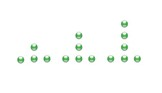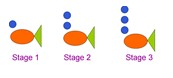# Finding patterns in shapes

#### Excel Pivot Tables and Dashboard. Simple & Quick tutorial!

Most Popular

7 Lectures 58 mins

#### Learn HTML Tables

15 Lectures 59 mins

#### Excel Analytics - Data Analysis with Pivot-Tables and Charts

46 Lectures 2.5 hours

In this lesson, we solve problems having series of shapes following some pattern.

We study and analyze the series of shapes to find the pattern or rule and based on that rule, we find the next or missing shape in the series.

The sequence of 3 figures shows a pattern. If the pattern repeats, how many marbles will there be in figure 5?### Solution

Step 1:

In the given pattern above, horizontal marbles are always 3 in number and vertical marbles in numbers are 1, 2, 3

Step 2:

So, figure 5 will have 3 horizontal marbles and 5 vertical marbles. A total of 3 + 5 = 8 marbles.

The sequence of figures shows a pattern. If the pattern repeats, how many bubbles will Stage 6 have?### Solution

Step 1:

The number of bubbles in the three stages are 1, 2, and 3. This is an arithmetic sequence whose common difference is 1.

Step 2:

As per the pattern, the stage 6 should have 3 + 1 + 1 + 1 or 6 bubbles.